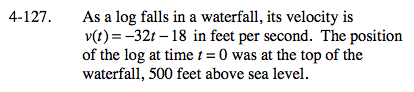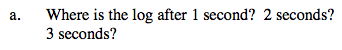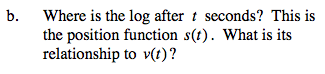### Home > CALC > Chapter 4 > Lesson 4.4.1 > Problem4-127

4-127.
1.As a log falls in a waterfall, its velocity is v(t) = −32t − 18 in feet per second. The position of the log at time t = 0 was at the top of the waterfall, 500 feet above sea level. Homework Help ✎

1. Where is the log after 1 second? 2 seconds? 3 seconds?

2. Where is the log after t seconds? This is the position function s(t). What is its relationship to v(t) ?You need to find the position function, s(t).

Antidifferentiate v(t).

Solve for C, by evaluating the given point s(0)=500.